# Thomas Winters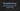# DeepStochLog

DeepStochLog is a neural-symbolic framework for neural stochastic logic programming. This allows to express background knowledge using logic and probabilities, and use this to more effectively train neural networks. It achieved state-of-the-art results on a wide variety of tasks, while scaling much better than alternative neural-symbolic frameworks.

For more in-depth, see the DeepStochLog Github repository and the DeepStochLog paper.

## Capabilities

### Sum of handwritten numbers

As an example consider having to calculate the sum of two handwritten numbers, but never having seen handwritten numbers with their numeric label, but only having seen having seen combinations of handwritten numbers together with their numerical sum.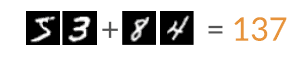Example of a sum of handwritten numbers

DeepStochLog encodes this information as follows:

``````digit_dom(Y) :- member(Y,[0,1,2,3,4,5,6,7,8,9]).

nn(number, [X], Y, digit_dom) :: digit(Y) --> [X].
1 :: addition(N) --> digit(N1),
digit(N2),
{N is N1 + N2}.``````

More generally, DeepStochLog can easily parse and classify sequences of subsymbolic information like images that follow a known grammar structure. It can even encode general problems like word algebra problems or labeling papers in a citation graph, or any other task that could be expressed using neural stochastic logic.

In our experiments in the paper, we found that for all tasks, DeepStochLog achieved state-of-the-art accuracy, but scaled better than other similar neural-symbolic frameworks.

## Defining Neural Definite Clause Grammars

Rules in DeepStochLog are modelled as grammar rules that are allowed to contain arguments, logic and (neural) probabilities.

``nn(m,[I1,…,Im],[O1,…,OL],[D1,…,DL]) :: nt --> g1, …, gn.``

Where:

• `nt` is an atom
• `g1,…,gn` are goals (goal = atom or list of terminals & variables)
• `I1,…,Im` and `O1,…,OL` are variables occurring in `g1,…,gn` and are the inputs and outputs of `m`
• `D1,…,DL` are the predicates specifying the domains of `O1,…,OL`
• `m` is a neural network mapping `I1,…,Im` to probability distribution over `O1,…,OL` (= over cross product of `D1,…,DL`)

## Understanding Neural Definite Clause Grammars

DeepStochLog extends definite clause grammars with neural probabilities to create "Neural Definite Clause Grammars". To understand this, let's take a look at the basic building blocks, namely context-free grammars and probabilistic context-free grammars, as well as it's logic-based extensions definite clause grammars and stochastic definite clause grammars.

### Context-free grammars

Context-free grammars model rules as just mappings from non-terminals to sequences of other non-terminals and terminals. It is useful for a wide variety of language tasks, such as:

• Finding out if a sequence is an element of the specified language
• Labeling the "part of speech"-tag of a terminal
• Generating all elements of language

### Probabilistic context-free grammars

Probabilistic context-free grammars extend context-free grammars by adding probabilities to every rule. The probabilities of all used rules in the expansion are then used to calculate the probability of a particular parse. This framework is useful for finding answers to the following questions:

• What is the most likely parse for this sequence of terminals? (useful for ambiguous grammars)
• What is the probability of generating this string?

### Definite clause grammars

Definite clause grammars extend context-free grammars with logic programming concepts. It allows for adding arguments to the non-terminals, and adding extra logic calculations between the curly brackets {}. Thanks to the addition of logic programming, this makes this type of grammar Turing-complete.

Definite clause grammars are useful for:

• Modelling more complex languages (e.g. context-sensitive)
• Adding constraints between non-terminals thanks to Prolog power (e.g. through unification)
• Extra inputs & outputs aside from terminal sequence (through unification of input variables)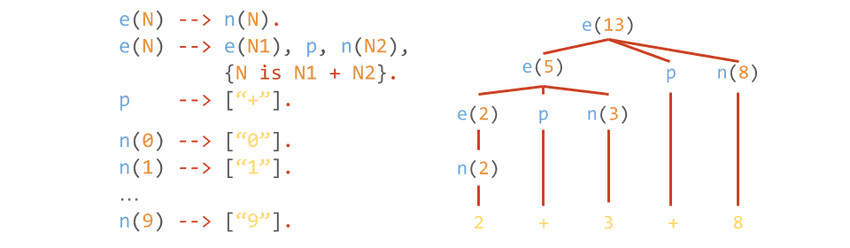Parsing using a definite clause grammars

### Stochastic definite clause grammars

Stochastic definite clause grammars add the same benefit to definite clause grammars as probabilistic context-free grammars add to context-free grammars, namely adding probabilities to rules. This thus gives rise to the same type of benefits, like finding the most likely parse. However, since expansions can fail in a definite clause grammar, probability mass can get lost in such a failing derivation, meaning that the sum of the probabilities of all possible elements is not necessarily 1.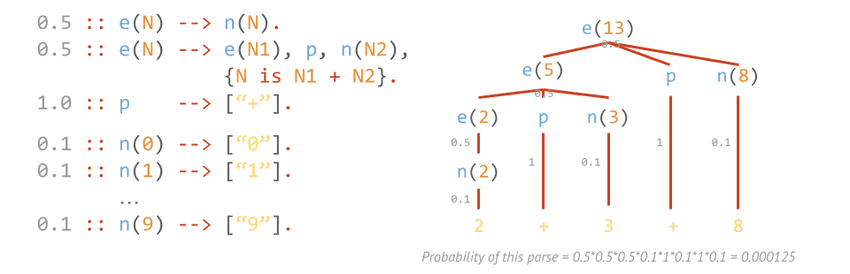Parsing using a stochastic definite clause grammars

### Neural definite clause grammars

Neural definite clause grammars add neural probabilities to stochastic definite clause grammars, which swap rule probabilities with neural networks that output a probability. This is the same trick as DeepProbLog does to probabilistic logic programming, but then to stochastic definite clause grammars.

This framework is useful for:

• Subsymbolic processing: e.g. tensors as terminals
• Learning rule probabilities using neural networks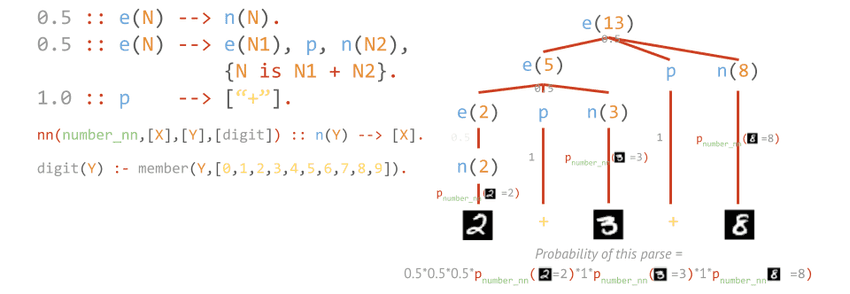Parsing using a neural definite clause grammars

## Paper

If you would like to refer to this work, or if use this work in an academic context, please consider citing the following paper:

``````@article{winters2021deepstochlog,
title={Deepstochlog: Neural stochastic logic programming},
author={Winters, Thomas and Marra, Giuseppe and Manhaeve, Robin and De Raedt, Luc},
journal={arXiv preprint arXiv:2106.12574},
year={2021}
}
``````

Or APA style:

Winters, T., Marra, G., Manhaeve, R., & De Raedt, L. (2021). Deepstochlog: Neural stochastic logic programming. arXiv Preprint arXiv:2106.12574.